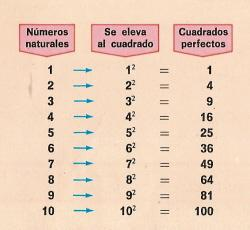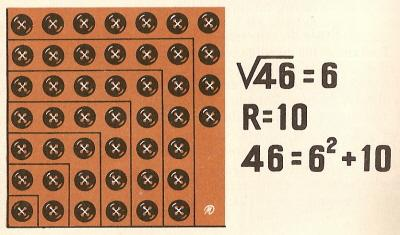Square root (1) to printName: ______________________________________  Subject: _______________________ Date: _______

Write on the right side what is missing.

1. concept of root

In the upper figure, we see the floor of a square room that has 100 tiles. How many tiles will the room have on each side? To solve this problem, we must found a number that when we raised it to the square is 100. The result is 10 because 10 x 10 = 100 , 102 = 100.

Therefore, the square root of 100 is 10.2. Elements of the root

The number 36 is the square of 6. We can also say that 6 is the square root of 36. The symbol  ų  is called radical sing.
In the previous example, 36 is called radicand, 6 is the square root and ų is the radical sign.

Exercises:

 1. In  ų4 = 2  the 2 is... 2. In  ų4 = 2  the  ų is... 3. In   ų4 =2 the 4 is... 4. In  ų16 = 4 the  ų is... 5. In   ų16 = 4 the 4 is... 6. In   ų16 =4 the 16 is...3. - Exact square root

When a natural number is raised to the power 2, we get the perfect squares. 36 is the perfect square of 6, we can also say that 6 is the square root of 36.

Exercises:

 1. The square root of 49 = 2. The square root of 81 = 3. The square root of 64 = 4. The square root of 100 = 5. The square root of 25 = 6. The square root of 36 =4. - Whole square root

If we want to find the square root of 46, we see that it is not a perfect square, since it is greater than 36 (62) and less than 49 (72). The root of 46 will have a whole part (6) and a decimal part.
The whole square root of a number is the root of the greatest perfect square root contained therein.
In this case the square of 6 (36) needs 10 to reach to 46. 46 -36 = 10.
The number 10 is called remainder

The remainder of the square root of a number is the difference between that number and the square of its whole square root.

You can see the topic of how to solve problems

Do these problems:

 1. In   ų26, the square root is... 2. In   ų26, the remainder is... 3. In   ų87, the remainder is... 4. In   ų87, the square root is... 5. In   ų50, the square root is... 6. In   ų50, the remainder is...

| Educational applications | Mathematics |
In Spanish  | Interactive

«Arturo Ramo GarcĒa.-Record of intellectual property of Teruel (Spain) No 141, of 29-IX-1999
Plaza Playa de Aro, 3, 1║ DO 44002-TERUEL